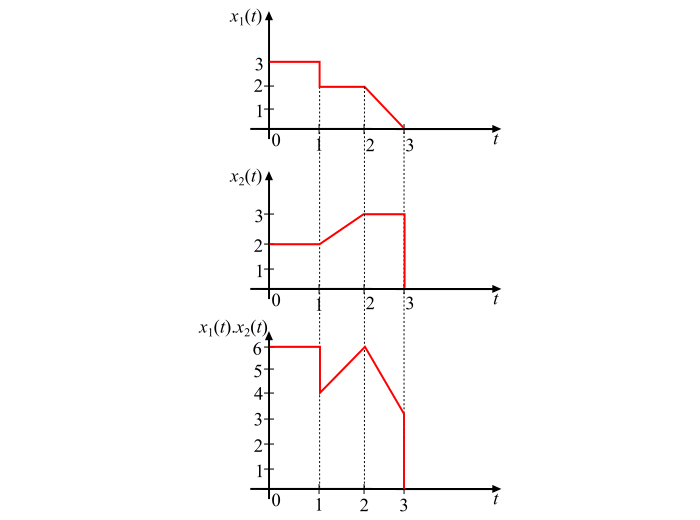# Signals and Systems: Multiplication of Signals

## Multiplication of Continuous-Time Signals

The product of two continuous-time signals can be obtained by multiplying their values at every instant of time. Consider two continuous time signals 𝑥1(𝑡) and 𝑥2(𝑡) as shown in the figure.## Explanation

The multiplication of the two signals can be performed by considering different time intervals as follows −

• For 𝟎 ≤ 𝒕 ≤ 𝟏: 𝑥1(𝑡) = 3 and 𝑥2(𝑡) = 2, thus

𝑥1(𝑡)𝑥2(𝑡) = 3 × 2 = 6

• For 1≤ 𝒕 ≤ 𝟐: 𝑥1(𝑡) = 2 and 𝑥2(𝑡) = 2 + (𝑡 − 1), hence,

𝑥1(𝑡)𝑥2(𝑡) = 2[2 + (𝑡 − 1)] = 4 + 4(𝑡 − 1)

• For 2≤ 𝒕 ≤ 𝟑: 𝑥1(𝑡) = 2 − (𝑡 − 2) and 𝑥2(𝑡) = 3, hence,

𝑥1(𝑡)𝑥2(𝑡) = [2 − (𝑡 − 2)]3 = 6 − 3(𝑡 − 2)

The multiplication of the signals (i.e., 𝑥1(𝑡)𝑥2(𝑡)) is shown in the figure.

## Multiplication of Discrete-Time Signals

The multiplication of two discrete-time signals 𝑥1(𝑛) and 𝑥2(𝑛) can be performed by multiplying the corresponding sample values.

Consider two discrete time sequences 𝑥1(𝑛) and 𝑥2(𝑛) as follows −

𝑥1(𝑛) = {−3, 1, 5, 1, 2}

𝑥2(𝑛) = {2, −1, 1, 3, −3}

Then, the product of these two signal is given by,

𝑥1(𝑡)𝑥2(𝑡) = {−3 × 2, 1 × (−1), 5 × 1, 1 × 3, 2 × (−3)}

⟹ 𝑥1(𝑡)𝑥2(𝑡) = {−6, −1, 5, 3, −6}